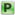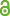Hauptmenü
• Autor
• Frisch, Sophie
• TitelPolynomial functions on upper triangular matrix algebras
• Datei
• DOI10.1007/s00605-016-1013-y
• Persistent Identifier
• Erschienen inMonatshefte für Mathematik
• Band184
• Erscheinungsjahr2017
• Heft2
• Seiten201-215
• ISSN1436-5081
• Zugriffsrechte• Download Statistik387
• Peer ReviewJa
• AbstractThere are two kinds of polynomial functions on matrix algebras over commutative rings: those induced by polynomials with coefficients in the algebra itself and those induced by polynomials with scalar coefficients. In the case of algebras of upper triangular matrices over a commutative ring, we characterize the former in terms of the latter (which are easier to handle because of substitution homomorphism). We conclude that the set of integer-valued polynomials with matrix coefficients on an algebra of upper triangular matrices is a ring, and that the set of null-polynomials with matrix coefficients on an algebra of upper triangular matrices is an ideal.